# 【python数据分析】数据的分组，遍历，统计

## 数据的分组，遍历，统计

### 1️⃣分组

Pandas提供了一个灵活高效的groupby功能，它使你能以一种自然的方式对数据集进行切片、切块、摘要等操作。

✨效果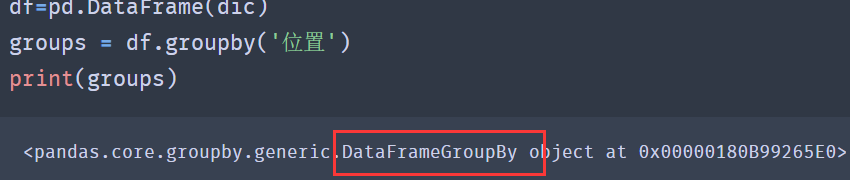``````from pandas import Series,DataFrame

lol_list = [['上单','Nuguri',31,78],
['打野','Tian',42,68],
['中单','Doinb',51,83],
['中单','Faker',38,76],
['辅助','Crisp',53,69]]

df = DataFrame(data=lol_list,
index=['a','b','c','d','e','f'],
columns=['位置','ID号','年龄','数据'])

groups = df.groupby('位置')
print('----------------------------------')

``````

✨效果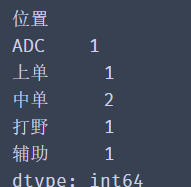[En]

According to the above method, we can get the proportion of each position. Don't hesitate to try it in the code box below.

``````for i,j in groups.size().items():
account = j/df.shape
dd="%.2f%%"%(account*100)
print(f"{i}占比为{dd}")
print(groups.size())
``````

✨效果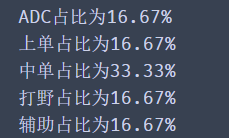df.groupby('位置')是根据位置列对整个数据进行分组， `&#x540C;&#x6837;&#x6211;&#x4EEC;&#x4E5F;&#x53EF;&#x4EE5;&#x53EA;&#x5BF9;&#x4E00;&#x5217;&#x6570;&#x636E;&#x8FDB;&#x884C;&#x5206;&#x7EC4;`，只保留我们需要的列数据。

``````group = df['年龄'].groupby(df['位置'])

print(group.groups)

print(group.get_group('中单'))
``````

✨效果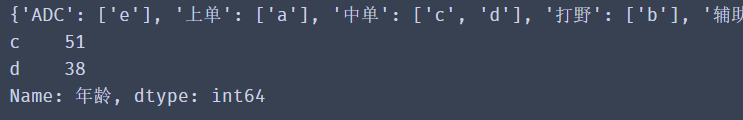1.代码 `group = df['&#x5E74;&#x9F84;'].groupby(df['&#x4F4D;&#x7F6E;'])`

2.上一步的代码也可改写成 `group = df.groupby(df['&#x4F4D;&#x7F6E;'])['&#x5E74;&#x9F84;']`

3.group.groups的结果是一个字典，字典的key是分组后每个组的名字，对应的值是分组后的数据，此方法方便我们查看分组的情况。

4.group.get_group('中单')这个方法可以根据具体分组的名字获取，每个组的数据

### 2️⃣对分组进行遍历

``````from pandas import Series,DataFrame

lol_list = [['韩国','上单','Nuguri',31,78],
['中国','打野','Tian',42,68],
['韩国','中单','Doinb',51,83],
['韩国','中单','Faker',38,76],
['中国','中单', 'xiye',21,45],
['中国','辅助','Crisp',53,69]]

df = DataFrame(data=lol_list,
index=['a','b','c','d','e','f','g'],
columns=['国家','位置','ID号','年龄','数据'])

group = df.groupby(df['位置'])['年龄']

print(group.groups)

print(group.get_group('中单'))

``````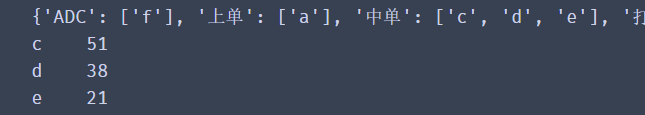[En]

Through the picture above, we can get the age of the middle order, so next we will calculate their average age and the maximum and minimum age (here the sample size is small, but does not affect the operation)

``````group = df.groupby('位置')

group_f = group.get_group('中单')
f_mean = group_f['年龄'].mean()
print(f_mean)

``````

✨效果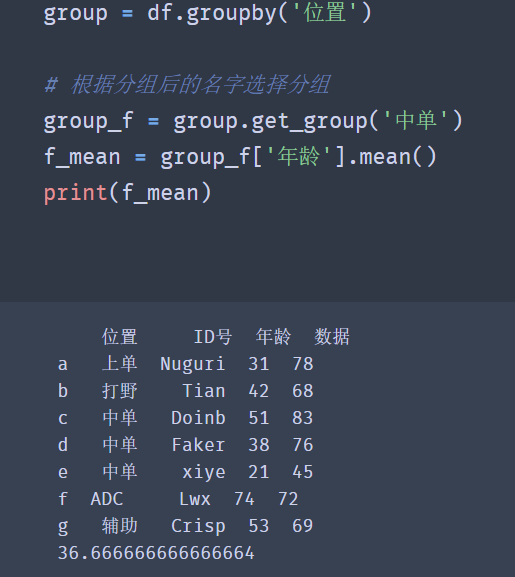🚩Pandas常用函数

[En]

The above code successfully calculates the data we want, and we can also traverse the grouped data and get their maximum age, minimum age and average age.

``````group = df.groupby('位置')

for i,j in group:
f_mean = j['年龄'].mean()
print(f"{i}的平均年龄为{f_mean}")

``````

✨效果### 3️⃣按多列进行分组

[En]

When our needs are more complex, if we want to divide a set of data on the basis of a corresponding data, for example, we need to get the number of locations of each country.

``````group = df.groupby(['国家','位置'])
df1=group.size()
print(df1)
``````

✨效果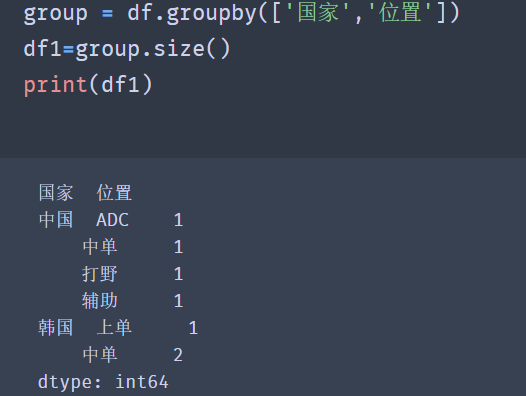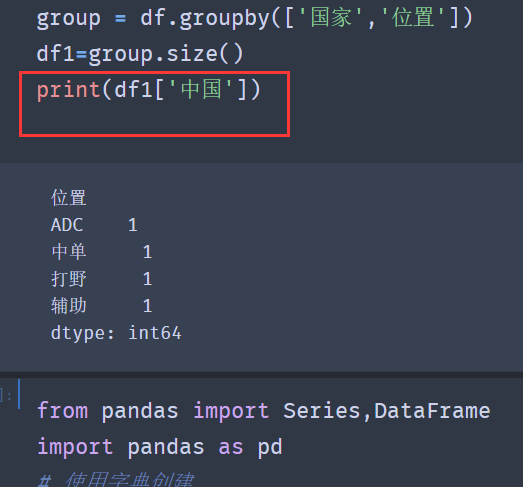### 4️⃣对分组后数据进行统计

[En]

Data statistics (also known as data aggregation) is the last step in data processing, usually to generate a single value for each array.

pandas提供了一个 `agg( )&#x65B9;&#x6CD5;`用来对分组后的数据进行统计。

``````import pandas as pd
groups = df.groupby('gender')
for group_name,group_df in groups:
f_se = group_df['age'].agg(['max','min','mean'])
print('{}组的最大年龄是{}，最小年龄是{}，平均年龄是{}'.format(group_name,f_se,f_se,f_se))

``````

🚩并且需要注意的是，如果是统计函数是pandas提供的，我们只需将函数的名字以字符串的形势存储到列表中即可，例如： `&#x5C06;max()&#x6539;&#x6210;'max'&#x3002;`

✨效果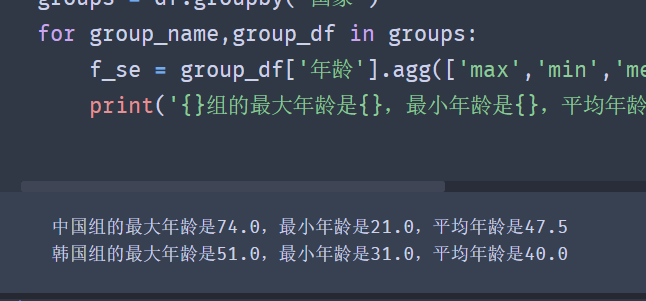``````def peak_range(df):
"""
返回数值范围
"""
return df.max() - df.min()
``````

``````groups = df.groupby('国家')
def peak_range(df):
"""
返回数值范围
"""
return df.max() - df.min()
for group_name,group_df in groups:
f_se = group_df['年龄'].agg(['max','min','mean',peak_range])
print(f'{group_name}组的最大年龄是{f_se}，最小年龄是{f_se}，平均年龄是{f_se},最大值减去最小值{f_se}')

``````

Original: https://blog.csdn.net/m0_53088614/article/details/121883360
Author: 弈鸣coding
Title: 【python数据分析】数据的分组，遍历，统计

# dirsearch安装+使用+运行问题（小宇特详解）

## 安装

dirsearch是一个用python开发的网站目录扫描工具
github下载地址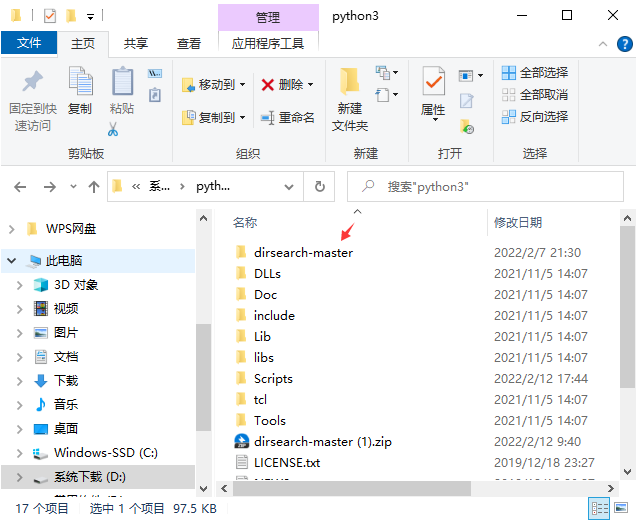## ; 使用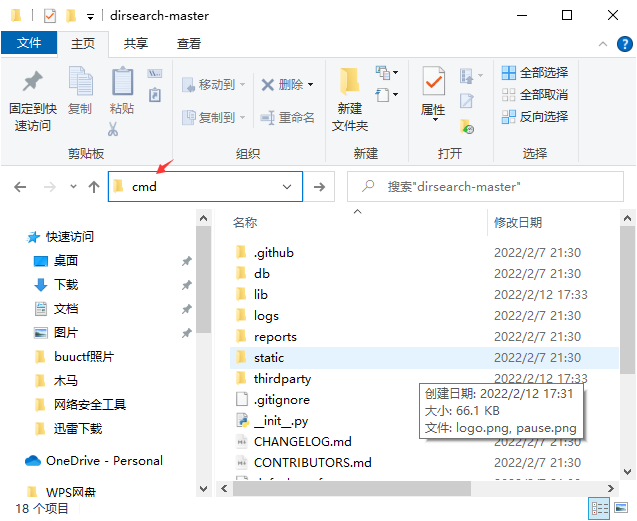``````python setup.py
``````

``````python dirsearch.py -u ip
``````

``````python dirsearch.py -h
``````

## 运行问题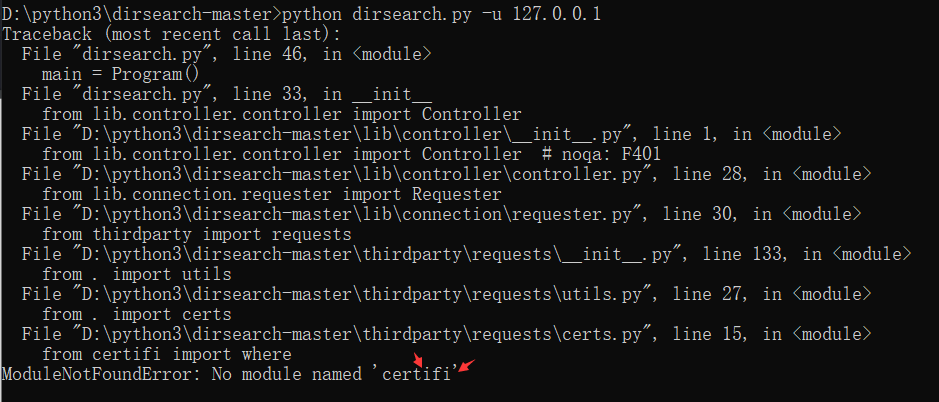``````pip install certifi
``````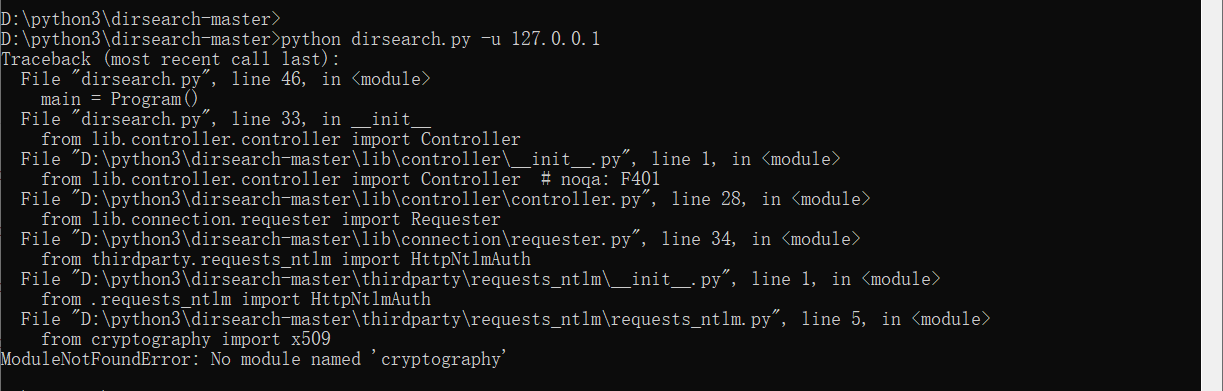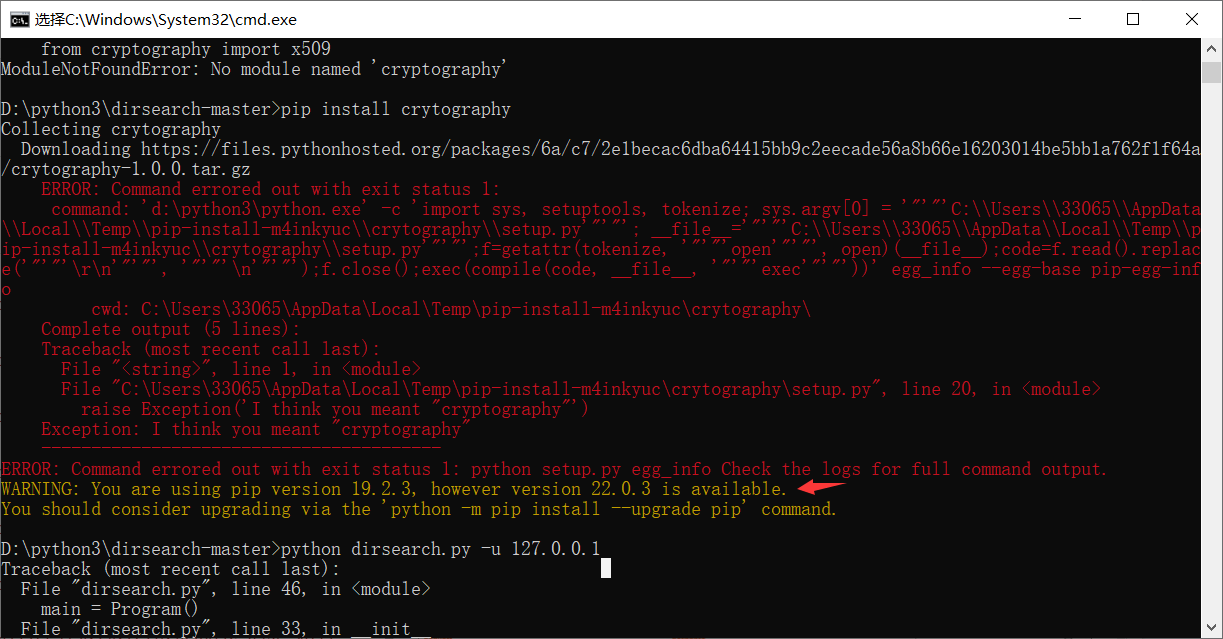``````pip -V
``````

``````D:\python3\python.exe -m pip install --upgrade pip
``````

``````pip install cryptography
``````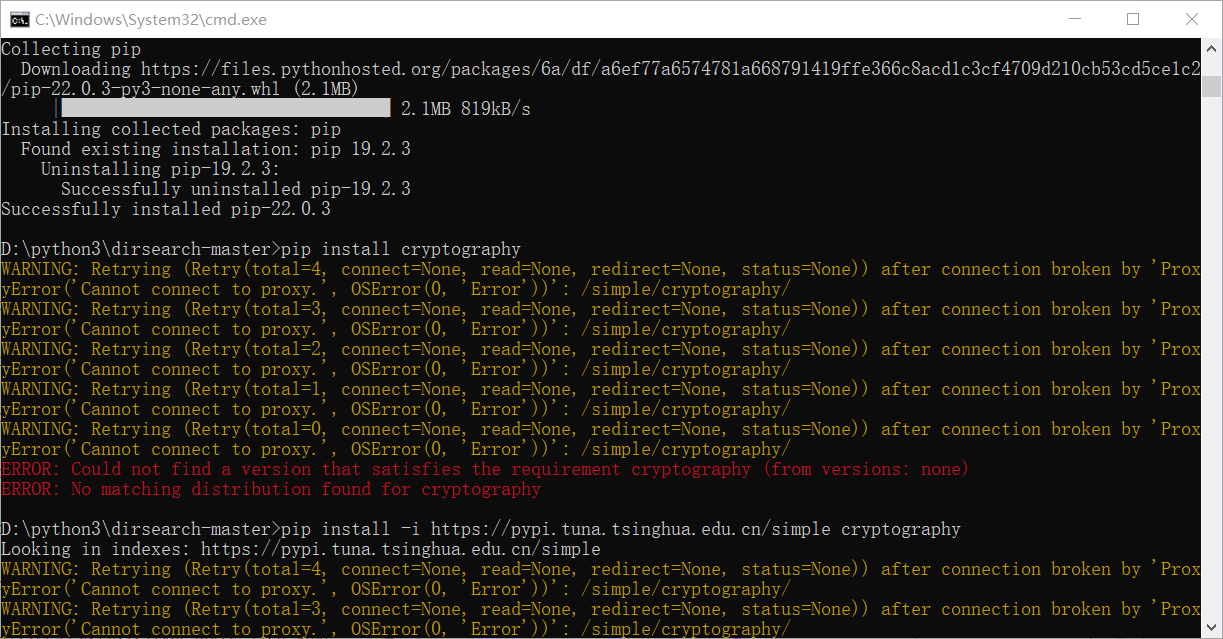### 希望我走过的坑你们不用再走。

Original: https://blog.csdn.net/xhy18634297976/article/details/122900500
Author: 小宇特详解
Title: dirsearch安装+使用+运行问题（小宇特详解）

## PINN(Python通过递归神经网络直接实现常微分方程积分)

Python 构建PINN ：（1 ）加载工具包；（2 ）搭建基于物理定律的模型结构；（3 ）将数据驱动内核与基于物理的内核耦合（PINN ）；（4 ）搭建神经网络；（5 ）使用训练好的神经网络预测 ...

## 深度学习之基于Tensorflow2.0实现VGG16网络

VGG系列的网络，在网络深度上相比于其之前的网络有了提升，VGG16与VGG19是VGG系列的代表，本次基于Tensorflow2.0实现VGG16网络。 1.VGG16 网络简介 VGG16网络模型...